# 7. Cosmology 1 Is the Universe Closed?

 Page 1/12 Date 26.04.2018 Size 0.57 Mb. #46938

## 7.1  Is the Universe Closed?

The unboundedness of space has a greater empirical certainty than any experience of the external world, but its infinitude does not in any way follow from this; quite the contrary. Space would necessarily be finite if one assumed independence of bodies from position, and thus ascribed to it a constant curvature, as long as this curvature had ever so small a positive value.

B. Riemann, 1854

Very soon after arriving at the final form of the field equations, Einstein began to consider their implications with regard to the overall structure of the universe.  His 1917 paper presented a simple model of a closed spherical universe which "from the standpoint of the general theory of relativity lies nearest at hand".  In order to arrive at a quasi-static distribution of matter he found it necessary to introduce the "cosmological term" to the field equations (as discussed in Section 5.8), so he based his analysis on the equations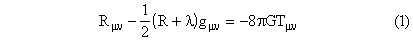where  is the cosmological constant.  Before invoking the field equations we can consider the general form of a metric that is suitable for representing the large-scale structure of the universe.  First, we ordinarily assume that the universe would appear to be more or less the same when viewed from the rest frame of any galaxy, anywhere in the universe (at the present epoch).  This is sometimes called the Cosmological Principle.

Then, since the universe on a large scale appears (to us) highly homogenous and isotropic, we infer that these symmetries apply to every region of space.  This greatly restricts the class of possible metrics.  In addition, we can choose, for each region of space, to make the time coordinate coincide with the proper time of the typical galaxy in that region.  Also, according to the Cosmological Principle, the coefficients of the spatial terms of the (diagonalized) metric should be independent of location, and any dependence on the time coordinate must apply symmetrically to all the space coordinates.  From this we can infer a metric of the form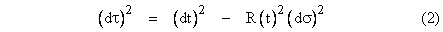where S(t) is some (still to be determined) function with units of distance, and d is the total space differential.  Recall that for a perfectly flat Euclidean space the differential line element is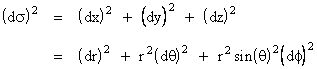where r2 = x2 + y2 + z2.  If we want to allow our space (at a given coordinate time t) to have curvature, the Cosmological Principle suggests that the (large scale) curvature should be the same everywhere and in every direction.  In other words, the Gaussian curvature of every two-dimensional tangent subspace has the same value at every point.  Now suppose we embed a Euclidean three-dimensional space (x,y,z) in a four-dimensional space (w,x,y,z) whose metric is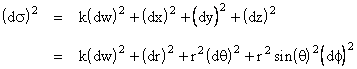where k is a fixed constant equal to either +1 or -1.  If k = +1 the four-dimensional space is Euclidean, whereas if k = -1 it is pseudo-Euclidean (like the Minkowski metric).  In either case the four-dimensional space is "flat", i.e., has zero Riemannian curvature.  Now suppose we consider a three-dimensional subspace comprising a sphere (or pseudo-sphere), i.e., the locus of points satisfying the condition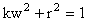From this we have w2 = (1  r2)/k = k  kr2, and therefore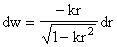Substituting this into the four-dimensional line element above gives the metric for the three-dimensional sphere (or pseudo-sphere)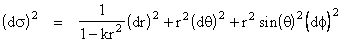Taking this as the spatial part of our overall spacetime metric (2) that satisfies the Cosmological Principle, we arrive at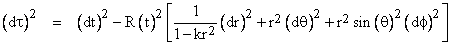This metric, with k = +1 and R(t) = constant, was the basis of Einstein's 1917 paper, and it was subsequently studied by Alexander Friedmann in 1922 with both possible signs of k and with variable R(t).  The general form was re-discovered by Robertson and Walker (independently) in 1935, so it is now often referred to as the Robertson-Walker metric.  Notice that with k = +1 this metric essentially corresponds to polar coordinates on the "surface" of a sphere projected onto the "equatorial plane", so each value of r corresponds to two points, one in the Northern and one in the Southern hemisphere.  We could remedy this by making the change of variable r  r/(1 + 3kr2), which (in the case k = +1) amounts to stereographic projection from the North pole to a tangent plane at the South pole.  In terms of this transformed radial variable the Robertson-Walker metric has the formAs noted above, Einstein originally assumed R(t) = constant, i.e., he envisioned a static un-changing universe.  He also assumed the matter in the universe was roughly "stationary" at each point with respect to these cosmological coordinates, so the only non-zero component of the stress-energy tensor in these coordinates is Ttt =  where  is the density of matter (assumed to be uniform, in accord with the Cosmological Principle).   On this basis, the field equations imply